# [Hardcore] Calculate a transfer funtion

#### akhu

Joined Jan 3, 2021
39
Good evening,

i asked this question in some german forums, but no one could help to explain. Maybe the english's can do.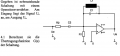How can I calculate the transfer function between UA/UE ?

The transfer function is defined as G(s)=UA/UE. And s is a substition of s=jw with w as the circular speed.

My fist idee a was a superposition:
$G(s)=G_1(s)+G_2(s)$
$G_1(s)=\frac{U_1}{U_e}$
$G_2(s)=\frac{U_a}{U_1}$

And U1 ist function which describe the voltage between C1 and R1.

Is it allowed to calculate like this ? Because I could not finde any Information about this.

Thanks for your help (sry for my broken english)

#### Papabravo

Joined Feb 24, 2006
20,142
Impedance of an inductor is $$j \omega L$$
Impedance of a capacitor is $$(j \omega C)^{-1}$$
Impedance of a resistor is $$R$$
It is just a matter of cranking out the algebra.

Last edited:

#### neonstrobe

Joined May 15, 2009
185
Good evening,

i asked this question in some german forums, but no one could help to explain. Maybe the english's can do. View attachment 227189
How can I calculate the transfer function between UA/UE ?
Note that the opamp gain can be written as A(V+ -V-). Set V+ to zero in this case. Are you given the open loop gain of the amplifier or is it supposed to be an ideal op-amp?
Use complex numbers for the impedances as PapaBravo mentioned and the TF should pop out.

#### akhu

Joined Jan 3, 2021
39
Impedance of an inductor is $$j \omega L$$
Impedance of a capacitor is $$(j \omega C)^{-1}$$
Impedance of a resistor is $R$
It is just a matter of cranking out the algebra.
Okay. But this not the point of matter. Because we can summ it up together with the Laplace variable to s.

Don't forget: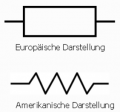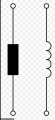Last edited:

#### akhu

Joined Jan 3, 2021
39
•click_here

#### Jony130

Joined Feb 17, 2009
5,445
We can treat this circuit as an inverting amplifier with the gain equal to:

$G(s) = - \frac{ Z_1(s)}{Z_2(s)}$

Where:

$Z_2(s) = R_2 + \frac{1}{s C_2} = \frac{1 + s R_2C_2}{s C_2}$

And

$Z_1(s) = L_1||C_1 = \frac{1}{\frac{1}{s L_1} + sC_2}=\frac{s L_1}{1 + s^2 L_1 C_1}$

Can you take it from here?

Last edited:
•akhu

#### akhu

Joined Jan 3, 2021
39
We can treat this circuit as an inverting amplifier with the gain equal to:

$G(s) = - \frac{ Z_1(s)}{Z_2(s)}$

Where:

$Z_2(s) = R_2 + \frac{1}{s C_2} = \frac{1 + s R_2C_2}{s C_2}$

And

$Z_1(s) = L_1||C_1 = \frac{1}{\frac{1}{s L_1} + sC_2}=\frac{s L_1}{1 + s^2 L_1 C_1}$

Can you take it from here?
I dont now but I belive your calculation is not correct. Or can you proof it ?

My calculation is in the attachment. But I'm also not sure.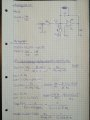Last edited:

#### Jony130

Joined Feb 17, 2009
5,445
Simply remove C1 and C2 from the circuit (short it out) and replace L1 with an R3 resistor. And find the voltage gain. And you will see that the R1 resistor doesn't have any influence on voltage gain as long as your amplifier is an Operational amplifier.

•akhu

#### akhu

Joined Jan 3, 2021
39
Okay the question ist, why does C1 and C2 doesn't have an impact on my circuit ? And with replace L1 to an resistor is this step allowed ? I mean L1 is complex and an resistor is real. And my secound question is it also possible to split the calculation of the transfer function in 2 steps ? Or should I calculate it in a whole huge step ?

#### Jony130

Joined Feb 17, 2009
5,445
Okay the question ist, why does C1 and C2 doesn't have an impact on my circuit ? And with replace L1 to an resistor is this step allowed ? I mean L1 is complex and an resistor is real.
This has nothing to do with your original circuit. Just to be able to quickly see why R1 has no influence on the gain try to solve this circuit: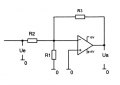And my secound question is it also possible to split the calculation of the transfer function in 2 steps ? Or should I calculate it in a whole huge step ?
For sure we can split the calculation of the transfer function. But the way you split the circuit is wrong and it will not give you the correct answer.

•akhu

#### akhu

Joined Jan 3, 2021
39
So, I tried it again. But If you told me the truht, I can't understand why C2 and C1 in my transfer function ? Or did I something wrong ? I trust you Jony, I hope that you dont tell me rubbish. Because I can show you an antoher example, which is similar to this. And I calculated it on the same way like this an my teacher said me, that is corrrect. So I'm very confused.

#### Jony130

Joined Feb 17, 2009
5,445
I can't understand why C2 and C1 in my transfer function ?
What?

Or did I something wrong ?
Why do you keep inserting R1 into equations? You haven't been taught, that U1 will be equal to 0V due to negative feedback action? Thus, no current will flow via the R1 resistor?
I'm going to sleep now.

•akhu

#### akhu

Joined Jan 3, 2021
39
What?

[ I meant, why C2 and C1 is in my final equation of the transfer function.]

Why do you keep inserting R1 into equations? You haven't been taught, that U1 will be equal to 0V due to negative feedback action? Thus, no current will flow via the R1 resistor?
I'm going to sleep now.
Honestly not. You are only one, but your explaination was good, now I understand it. Hmm, so the only thing I got is R2 and R3 right ? So last thing what I can't understand is why I can neglect the capacities C1 and C2 ?

Maybe some can show me the final function. I guess this would be the best way.

#### akhu

Joined Jan 3, 2021
39
Furthermore:

The next questions are:

1.) What is the reaction for high & low frequencies ? ( s -> inf & s->0)

2.) And what is the problem with the frequency of:$s=\frac{j}{\sqrt{L1C_1}}$

I hope some has an idea.

#### Jony130

Joined Feb 17, 2009
5,445
Honestly not. You are only one, but your explaination was good, now I understand it. Hmm, so the only thing I got is R2 and R3 right ?
Here is the circuit:
View attachment 227209

Are you able to find the voltage gain for such a simple circuit?

1.) What is the reaction for high & low frequencies ? ( s -> inf & s->0)
But we can answer this just by looking at your circuit. We don't need to know the transfer function.

For s -> 0 we have Xc = ∞, XL = 0Ω

and for s ->∞ we have Xc = 0Ω, XL = ∞

2.) And what is the problem with the frequency of:$s=\frac{j}{\sqrt{L1C_1}}$
Do you know how an LC circuit behavior at resonance?

•akhu

#### akhu

Joined Jan 3, 2021
39
Here is the circuit:
View attachment 227209

Are you able to find the voltage gain for such a simple circuit?

But we can answer this just by looking at your circuit. We don't need to know the transfer function.

For s -> 0 we have Xc = ∞, XL = 0Ω

and for s ->∞ we have Xc = 0Ω, XL = ∞

Do you know how an LC circuit behavior at resonance?
Yes, the voltage gain for this simple circuit should be:

$G(s)=-\frac{R_3}{R_2}$

with:$R_3(s)=( \frac{1}{sL_1} +sC_1)^-1$

and R2 with:

$R_2(s)= R_2+\frac{1}{sC_2}$

And R1 doesn't have an impact, because there is not a current flow right ?

So my final transfer function muste be in the orginal case: $G(s)=-\frac{sL_1}{\frac{1+s^2L_1C_1}{sC_2}+R_2+s^2L_1C_1R_2}$

And the sign of '' - ''is, because the the opamp has also a minus at the port above right ?

----------------------
For s -> 0 we have Xc = ∞, XL = 0Ω

and for s ->∞ we have Xc = 0Ω, XL = ∞

Ohh realy, and what is so the effect on the gain ? Does we have a better gain on s-> oder does we have a better gain on s -> 0 ?

-------------------------
Do you know how an LC circuit behavior at resonance?

Not realy, I know what an LC circuit is. But the the behavior at resonance. Maybe, that the gain is going to zero ?

#### akhu

Joined Jan 3, 2021
39
An LC circuiut behavior at resonance is, that the energry between the L & C is

exchanged periodically. So the gain must be zero, because the energy is locked up between L & C right ?

But formular for the resonace frequency is:

$f_0=\frac{1}{2\pi\sqrt(LC)}$

And what is now the contex to:

$s=\frac{j}{\sqrt{L1C_1}}$

Last edited:

#### akhu

Joined Jan 3, 2021
39
Thanks a lot for your engagment Jony130. So the peak of the magnitue curve and the falling of frequency curve must be the resonance frequency of my LC circuit right ?

But the task was, what is the problem with accompanied wiht the frequency of

$s=\frac{j}{\sqrt{L1C_1}}$

In other word. What kind of a problem is resulting with that frequency for my circiut ?

As far as I could see this circiut is a low pass filter. Because low frequncy can pass, and high frequceny is going be filtred. But please correct me if I am wrong.

And an another thing what is confusing me, that I can't which case this is.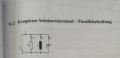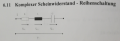Because L1 and C1 parraell. But the Resistor isnt parralel. Which case does we have on the orginal circut above ?

And the next one what is the differnces between the behaviors of:

series oscillation circuit to parallel resonant circuit ?

Last edited: